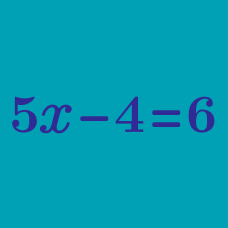Algebra

# Multi-Step Equations

What is the value of $x$ that satisfies the equation $\frac{x-21}{7}=3?$

What is the value of $x$ that satisfies the equation $2x-17=29?$

What is the value of $x$ that satisfies $\frac{1}{11}x+1=\frac{1}{4}x+\frac{4}{11}$?

What is the value of $x$ that satisfies the equation $x-17=\frac{1}{2}(x-8)+5?$

What is the value of $x$ that satisfies the equation $5(x-1)=4x+7$?

×

Problem Loading...

Note Loading...

Set Loading...